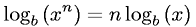Equations > Algebra > Logarithms > Logarithm of an Exponential

### Logarithm of an ExponentialLatex Code:

MathML Code:

 ${\mathrm{log}}_{b}\left({x}^{n}\right)=n{\mathrm{log}}_{b}\left(x\right)$

MathType 5.0: